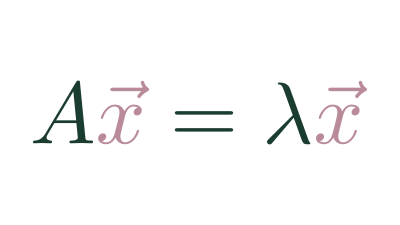## EigenvectorAn Eigenvectors of a Matrix Transformation is any non-zero vector that remains on its Vector Span after being transformed.

That means that performing the transformation is equivalent to scaling the vector by some amount. The amount it scales the Eigenvector is called the Eigenvalue.

For example, if we transform the basis vectors with matrix $\begin{bmatrix}2 && 1 \\ 0 && 2\end{bmatrix}$, we can see that $\hat{j}$ is knocked off its span, where $\hat{i}$ is simply scaled by 2.

One other particular case of vector that remains on its span is the zero vector: $\vec{v}=\begin{bmatrix}0\\0\end{bmatrix}$, but that's not an Eigenvector.

When performing 3d rotations, the Eigenvectors are particularly useful as they describe the axis of rotation.

The notation for describing the relationship between the matrix transformation of the vector and same scaling quality equivalent:

$A\vec{v} = \lambda\vec{v}$